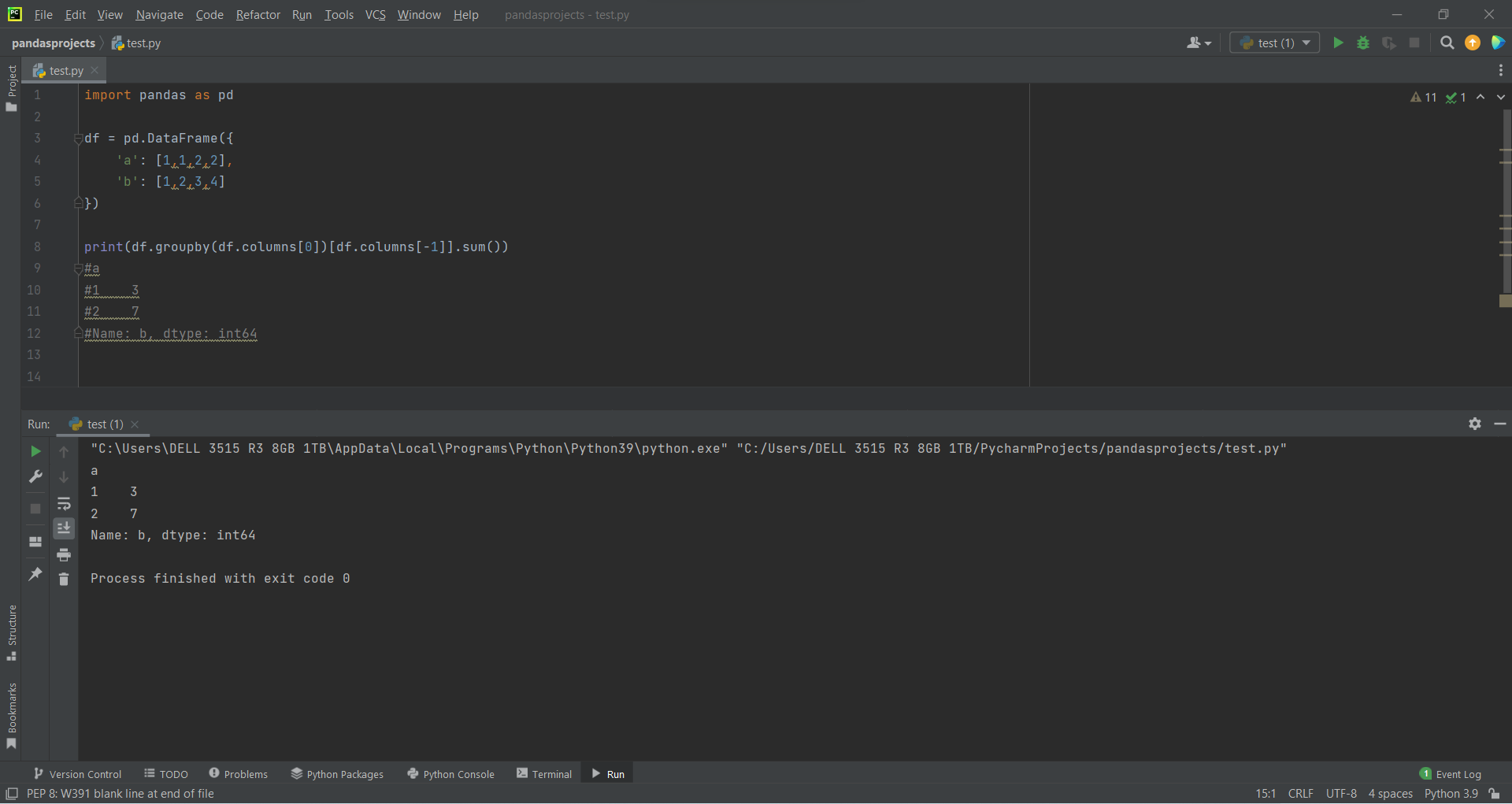# Group a DataFrame by Column using Pandasby Abdul Rawoof A RUpdated: Feb 28, 2023Solution Kit

Grouping a DataFrame by column using Pandas is a common operation in data analysis. It involves splitting a dataset into groups based on the unique values in a specific column and then applying a function to each group.

This grouping operation is useful for various tasks such as:

• Aggregating data - Grouping a DataFrame allows you to aggregate data by a particular column. You can apply different aggregation functions like sum(), mean(), min(), max(), count(), etc., to the groups and summarize the data for each unique value in the column.
• Data Exploration - Grouping a DataFrame allows you to explore the relationships between different variables in the dataset. Using plots and charts, you can group the data based on different columns and visualize the relationships between the groups.
• Data Cleaning - Grouping a DataFrame allows you to identify and clean data that contains errors, inconsistencies, or missing values. You can group the data by a particular column and then apply filtering and data-cleaning techniques to the groups with issues.

groupby() is a method in the Pandas library for grouping a DataFrame by one or more columns. It is a powerful tool for data aggregation and analysis. The groupby() method splits the data into groups based on the unique values in one or more columns. Then, an aggregation function, such as sum(), mean(), min(), max(), count(), etc., is applied to each group to summarize the data.

Grouping a DataFrame by column using Pandas is a powerful technique for exploring, summarizing, and cleaning datasets. It helps you to gain insights into your data and make better-informed decisions.

Here is an example of how to Group a DataFrame by Column using Pandas:Fig : Preview of the output that you will get on running this code from your IDE.

### Code

In this solution we're using Pandas library.

### Instructions

Follow the steps carefully to get the output easily.

2. Create a new file(eg.test.py).
3. Copy the snippet using the 'copy' and paste it in that python file in your IDE.
4. Import Pandas library by using the command - "import pandas from pd".
5. Add print at end of the line(refer preview of the output for your reference).
6. Run the file to generate the output.

I hope you found this useful. I have added the link to dependent library, version information in the following sections.

I found this code snippet by searching for 'pandas dataframe groupby column' in kandi. You can try any such use case!

### Environment Tested

I tested this solution in the following versions. Be mindful of changes when working with other versions.

1. The solution is created in PyCharm 2021.3.
2. The solution is tested on Python 3.9.7.
3. Pandas version-v1.5.2.

Using this solution, we are able to group a dataframe by column using pandas with simple steps. This process also facilities an easy way to use, hassle-free method to create a hands-on working version of code which would help us to group a dataframe by column using pandas.

### Dependent Library

pandasby pandas-dev

Python37415Version:v2.0.0rc1Flexible and powerful data analysis / manipulation library for Python, providing labeled data structures similar to R data.frame objects, statistical functions, and much more

Support
Quality
Security
Reuse

pandasby pandas-dev

Python37415Version:v2.0.0rc1License: Permissive (BSD-3-Clause)

Flexible and powerful data analysis / manipulation library for Python, providing labeled data structures similar to R data.frame objects, statistical functions, and much more
Support
Quality
Security